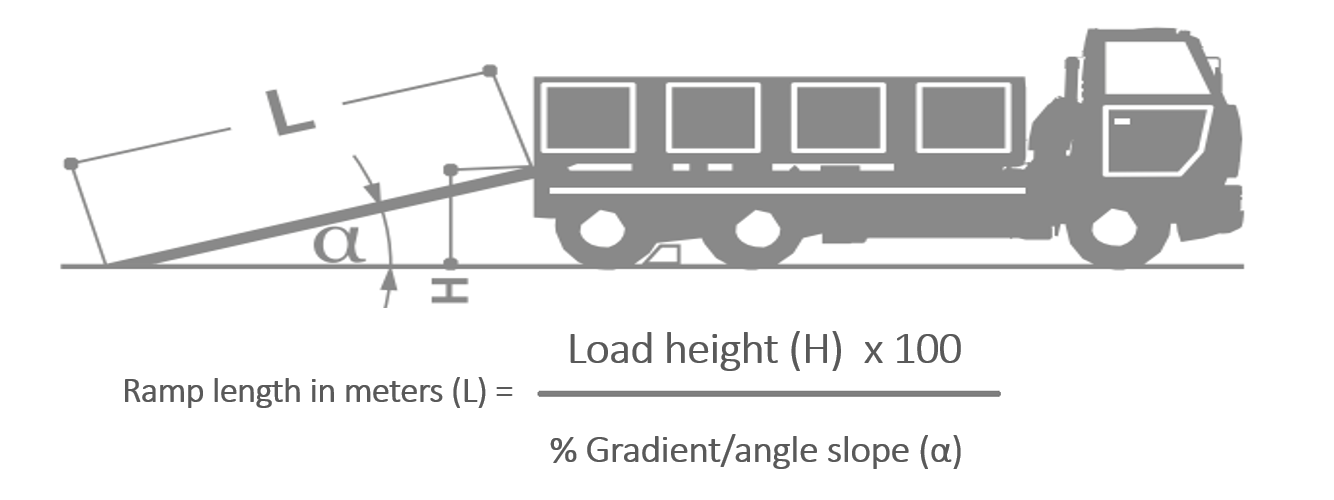# Plant Machinery Ramp Length Calculator

### Ramp length

Our guide makes it easy to calculate the length of ramp required for loading ramps. Alternately you can use our own ramp length calculator which is further down the page.

The slope of the ramps during use should not exceed a maximum gradient of 30%, which is equal to 16.5°. In order to determine the minimum length of the ramps, apply the below formula:Example:

Let's assume we need to overcome a load height (H) equal to 1.20 meters, with the load height (H) being the overall vertical height from the ground up to the bed of the vehicle where the ramp will rest.

By applying the formula indicated above, we get the following:
RAMP LENGTH IN METRES (L) = 1.20 metres x 100 / 30 = 4 metres long ramp required.

You can use the above formula to calculate the ramp length required or alternately please type your load height and the gradient "30" into our length calculator below: